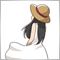# Custom EMA383

Hi,

I try to create multiple EMAs which is parallel to H1 Ema 20 with 10 pips interval by editing the custom moving average as follow (highlighted ) and it doesnt create parallel lines but overlap on existing ema 20 line. The completed file is attached. Please help.

```//+------------------------------------------------------------------+

//|  exponential moving average                                      |

//+------------------------------------------------------------------+

void CalculateEMA(int rates_total,int prev_calculated,const double &price[])

{

int    i,limit;

double SmoothFactor=2.0/(1.0+InpMAPeriod);

//--- first calculation or number of bars was changed

if(prev_calculated==0)

{

limit=InpMAPeriod;

ExtLineBuffer=price;

for(i=1; i<limit; i++)

ExtLineBuffer[i]=price[i]*SmoothFactor+ExtLineBuffer[i-1]*(1.0-SmoothFactor)+InpMAUpDown/100;

}

else

limit=prev_calculated-1;

//--- main loop

for(i=limit; i<rates_total && !IsStopped(); i++)

ExtLineBuffer[i]=price[i]*SmoothFactor+ExtLineBuffer[i-1]*(1.0-SmoothFactor)+InpMAUpDown/100;

//---

}```
Files:20210

chua le: and it doesnt work.
1. When you post code please use the CODE button (Alt-S)! (For large amounts of code, attach it.) Please edit your post.
General rules and best pratices of the Forum. - General - MQL5 programming forum
Messages Editor

2. "Doesn't work" is meaningless - just like saying the car doesn't work. Doesn't start, won't go in gear, no electrical, missing the key, flat tires - meaningless. There are no mind readers here and our crystal balls are cracked.

3. Did you set the two arrays to not as series, as that is how you're using them?524

Complete calculation of the EMA and next add the UpDown. Otherwise, it affects the calculation of the next EMA.
What is "/100"? Usually adjust the value with Point ().383

Hi ,

Thanks. "/100" is Multiplier for different decimal points. I will replace it with a interger variable.  I am not sure where shall I add the UpDown, could you please helping?

```//+------------------------------------------------------------------+

//|  exponential moving average                                      |

//+------------------------------------------------------------------+

void CalculateEMA(int rates_total,int prev_calculated,const double &price[])

{

int    i,limit;

double SmoothFactor=2.0/(1.0+InpMAPeriod);

//--- first calculation or number of bars was changed

if(prev_calculated==0)

{

limit=InpMAPeriod;

ExtLineBuffer=price;

for(i=1; i<limit; i++)

ExtLineBuffer[i]=price[i]*SmoothFactor+ExtLineBuffer[i-1]*(1.0-SmoothFactor)+InpMAUpDown/100;

}

else

limit=prev_calculated-1;

//--- main loop

for(i=limit; i<rates_total && !IsStopped(); i++)

ExtLineBuffer[i]=price[i]*SmoothFactor+ExtLineBuffer[i-1]*(1.0-SmoothFactor)+InpMAUpDown/100;

//---

}```524

```for(i=limit; i<rates_total && !IsStopped(); i++)
ExtLineBuffer[i]=price[i]*SmoothFactor+ExtLineBuffer[i-1]*(1.0-SmoothFactor);

for(i=limit; i<rates_total && !IsStopped(); i++)
ExtLineBuffer[i]+=InpMAUpDown*Point()*10;```383

Thank you ALL, it works now